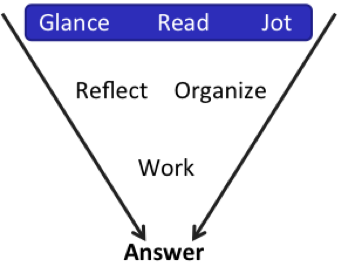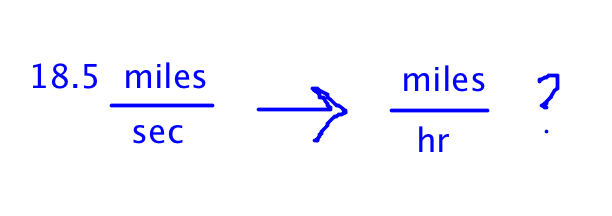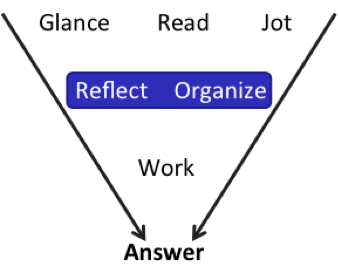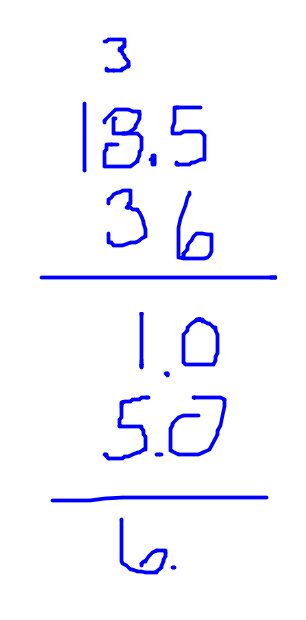More Fast Math for the GMAT – Part 7:

by on August 7th, 2017A while back, we started a series on Fast Math for the GMAT—here’s the link if you want to start from the beginning.

In our last installment, I gave you two problems to try. We’ve already discussed the first one; here’s the second one again (from the free problem set that comes with GMATPrep®).

“The Earth travels around the Sun at a speed of approximately 18.5 miles per second. This approximate speed is how many miles per hour?

“(A) 1,080

“(B) 1,160

“(C) 64,800

“(D) 66,600

“(E) 3,996,000”

What do you think?Those answers … they are seriously far apart. The first two are “clumped,” then the next two, and that last one is totally different.

We’re definitely going to estimate on this one.Also, just from glancing at the answers, I’m guessing that the correct answer will not be (E). Chances are pretty good that the correct answer will have another “close” answer based on making some small mistake—or based on making the problem just a little harder to estimate.

Jot down the details.Where should we start?How do you go from seconds to hours?

60 seconds → 1 minute

60 minutes → 1 hour

So we need 60 twice. Are we … multiplying? Dividing? Glance at the answers again or think it out logically. All of the answers are greater than 18.5, so I must have to multiply. And logically, that makes sense: If I go 18.5 miles in one second, then I should be able to go a lot further in a whole hour.

Okay, now which “clump” of answers is the right clump? 18.5 is a really annoying number, but the clumps are so far apart that I can just call that 20 for now.

(20)(60) = 1,200

Hmm. I still need to multiply by another 60, so answers (A) and (B) are out.

But I’m only multiplying by another 60, so answer (E) is out, too. It’s down to (C) and (D).

If I just multiply by another 60 now…will it be close enough? I’m not sure. I overestimated (from 18.5 to 20) and the two final numbers are pretty close. If the answer is (C), I might think it’s (D) just from the error I introduced in my estimation.

In fact, (1,200)(60) = 72,000. Definitely not good enough. Okay, what should I do next?

The math that actually needs to happen is this:

(18.5)(60)(60)

The latter two are easy: 3,600. It’s that 18.5 that’s the problem. I could just do long multiplication at this point…but long multiplication is so annoying! Also, I just noticed something else.

The final two answers are 64,800 and 66,600. Often, on problems like this, there’s a way to calculate just the units digit and use that to answer … but on this problem, the units digits are the same. SO annoying.

BUT! I can actually change the problem so that the units digits are different. Here’s how.

Again, here’s the “official” math that needs to happen and here are the two answers:

(18.5)(60)(60)

(C) 64,800

(D) 66,600

And here’s how I’m going to change the problem:

(18.5)(6)(6)

(C) 64,8

(D) 66,6

Wait, what did I just do?I got rid of the two zeros. So now, I just have to figure out what the units digit of (18.5)(36) is—6 or 8?The units digit is 6, so the answer is (D).

Look at that math carefully to see what I did—and did not—do. First, I multiplied the 6 by the 5, and carried the 3. Next, I multiplied the 6 by the 8, added the 3 … and then only wrote down the units digit. (6)(8) + 3 = 51, but I only wrote down the number 1. Then I stopped working on that line. How did I know that was as far as I needed to go?

Because the number 18.5 has one decimal, I knew that the first number I calculated (which turned out to be 0) would be a decimal, too—the tenths place. The next number to the left will become part of the units digit. I don’t care about more than the units digit, so I don’t have to keep calculating.

I do have to go to the next number in 36, the 3, to see what that contributes to the units digit. First, at the second step in long multiplication, go down one line and automatically start off with a 0 in what will become the tenths place. Then, multiply the 3 and the 5 to get 15—but, again, I care only about the units digit, so I only wrote down the 5.

Finally, 1 + 5 = 6, so the answer must be 66,6 (or 66,600, once I add the zeros back in). Done!

Key Fast Math Takeaways:

(1) If you think you need to do long division or long multiplication, stop for a moment. Reflect. Even if you have to do partial long division or multiplication, how much do you really have to do?

(2) As we discussed last time, don’t start solving on PS until you’ve looked at those answer choices! Sometimes, they contain very important clues about the most efficient way to solve.

* GMATPrep® questions courtesy of the Graduate Management Admissions Council. Usage of this question does not imply endorsement by GMAC.